\u200E2022年5月飞桨框架2.3版本正式发布，相比飞桨框架2.2版本，API体系更加丰富，新增了100多个API，覆盖自动微分、概率分布、稀疏Tensor、拟牛顿优化器、线性代数、框架性能分析、硬件设备管理、视觉和语音领域等方面。整体上进一步丰富了飞桨框架动静统一、高低融合的API体系。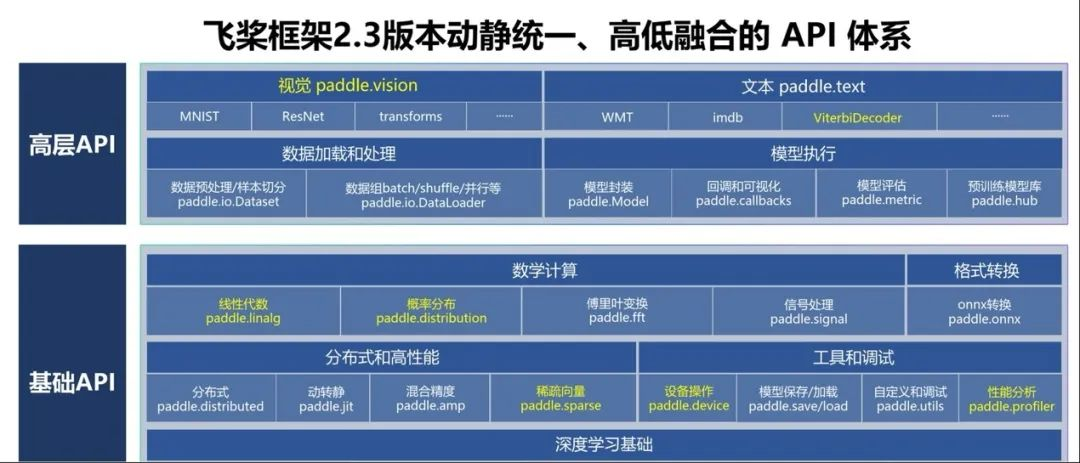1

﻿﻿Jacobian(func, xs)﻿计算﻿func﻿在 ﻿xs﻿处的雅可比矩阵。为了降低计算整个矩阵的性能和显存开销，实现上采用了延迟计算方式，即按需计算部分行，并进行缓存。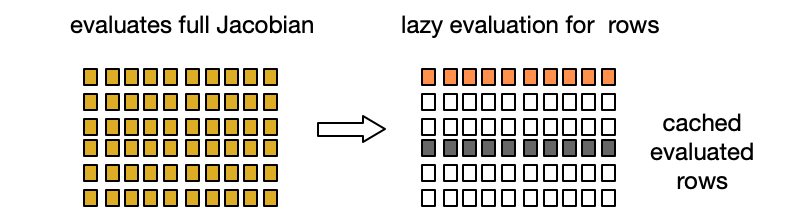`import paddledef func(x, y):    return paddle.matmul(x, y)x = paddle.to_tensor([[1., 2.], [3., 4.]])J = paddle.incubate.autograd.Jacobian(func, [x, x])print(J[:, :])# Tensor(shape=[4, 8], dtype=float32, place=Place(gpu:0), stop_gradient=False,#        [[1., 3., 0., 0., 1., 0., 2., 0.],#         [2., 4., 0., 0., 0., 1., 0., 2.],#         [0., 0., 1., 3., 3., 0., 4., 0.],#         [0., 0., 2., 4., 0., 3., 0., 4.]])print(J[0, :])# Tensor(shape=, dtype=float32, place=Place(gpu:0), stop_gradient=False,#        [1., 3., 0., 0., 1., 0., 2., 0.])print(J[:, 0])# Tensor(shape=, dtype=float32, place=Place(gpu:0), stop_gradient=False,#        [1., 2., 0., 0.])`
﻿﻿﻿Jacobian延迟计算示例

Hessian(func, xs)﻿计算﻿func﻿在﻿xs﻿的海森矩阵，﻿func﻿要求是﻿vector-scalar﻿函数，即输出是单个元素。海森矩阵是输出对输入的二阶导数，也可以理解为雅可比矩阵对输入的导数，因此实现上基于﻿Jacobian﻿，同样支持延迟计算与缓存。

``def _jac_func(*xs):    jac = Jacobian(func, xs, is_batched=is_batched)    if (is_batched and jac.shape != 1) or (not is_batched and                                              jac.shape != 1):        raise RuntimeError(            "The function given to Hessian shoud return as single element Tensor or batched single element Tensor."        )    return jac[:, 0, :] if is_batched else jac[0, :]self.symbolic = Jacobian(_jac_func, xs, is_batched=is_batched)``

Hessian实现核心代码

﻿﻿jvp(func, xs, v）﻿即雅可比矩阵-向量乘积，计算函数﻿func﻿在﻿xs﻿处的雅可比矩阵与向量﻿v﻿乘积。﻿jvp﻿主要用途是计算前向微分，前向微分即依据链式法则，从前向后遍历计算图，计算当前变量对输入的微分。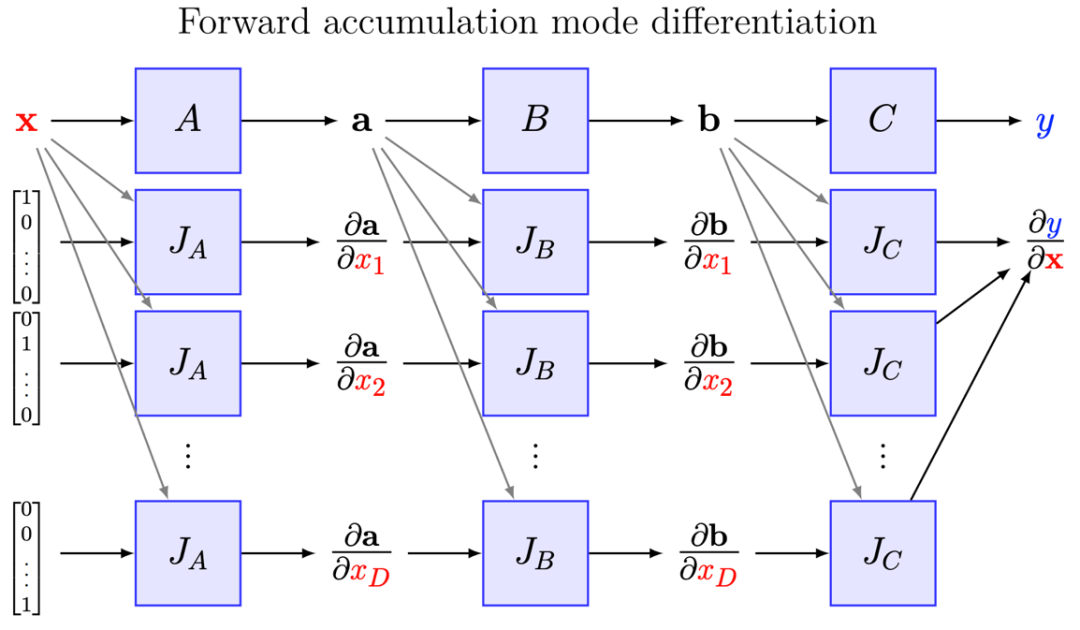﻿﻿﻿vjp(func, xs, v）﻿即向量-雅可比矩阵乘积，计算向量﻿v﻿与函数﻿func﻿在﻿xs﻿处的雅可比矩阵乘积。﻿vjp﻿主要用途是计算反向微分，反向微分即依据链式法则，从后向前遍历计算图，计算输出对当前变量的微分。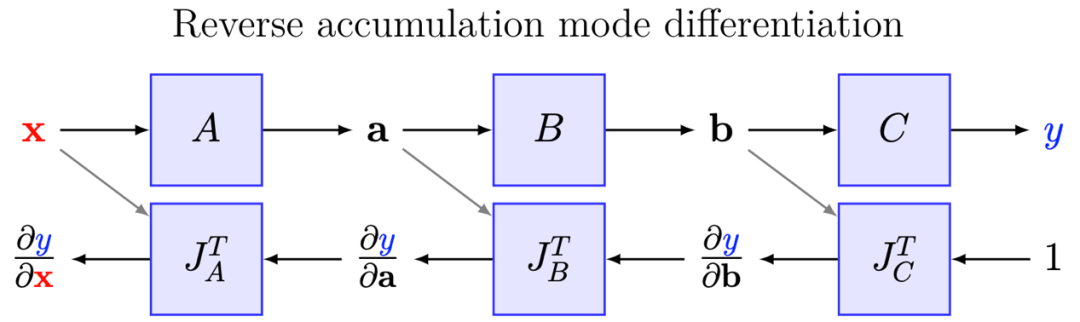﻿

2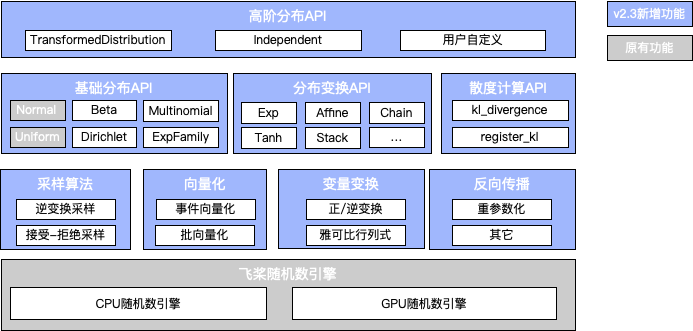﻿

（1）概率统计与随机采样

``import paddle beta = paddle.distribution.Beta(0.5, 0.5)samples = beta.sample((10000,))``

Beta 分布采样示例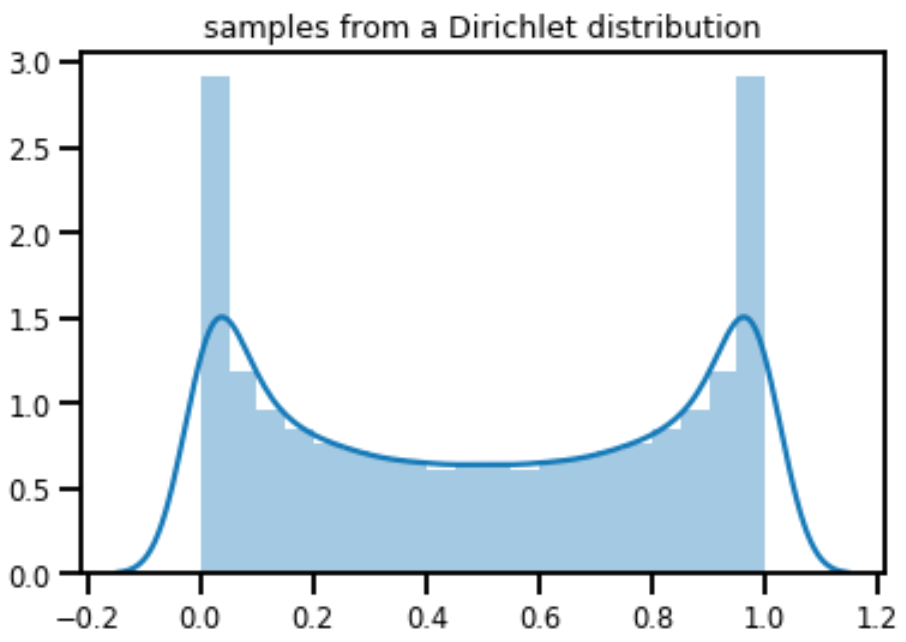﻿

（2）分布变换

``import paddleimport matplotlib.pyplot as pltimport numpy as npimport seaborn as snsnormal = paddle.distribution.Normal(0., 1.)samples = normal.sample()sns.distplot(samples)plt.title('samples from a Norma(0., 1.)')plt.show()affine = paddle.distribution.AffineTransform(paddle.to_tensor(2.), paddle.to_tensor(2.))transformed_samples = affine.forward(samples)sns.distplot(transformed_samples)plt.title('samples from a AffineTransform(2., 2.) Normal(0., 1.)')plt.show()``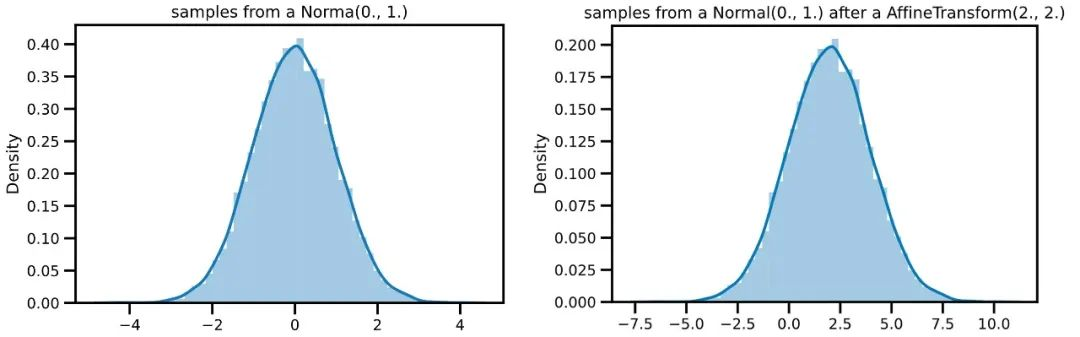（3）统一KL散度计算

``import paddlep = paddle.distribution.Beta(alpha=0.5, beta=0.5)q = paddle.distribution.Beta(alpha=0.3, beta=0.7)print(paddle.distribution.kl_divergence(p, q))# Tensor(shape=, dtype=float32, place=CUDAPlace(0), stop_gradient=True,#        [0.21193528]``

（4）构建高阶分布

``import paddle # 基于标准正态分布和仿射变换，构建新的高阶分布d = paddle.distribution.TransformedDistribution(    paddle.distribution.Normal(0., 1.),     [paddle.distribution.AffineTransform(paddle.to_tensor(1.), paddle.to_tensor(2.))])print(d.sample())# Tensor(shape=, dtype=float32, place=Place(gpu:0), stop_gradient=True,#        [-0.10697651,  3.33609009, -0.86234951,  5.07457638,  0.75925219,#         -4.17087793,  2.22579336, -0.93845034,  0.66054249,  1.50957513])print(d.log_prob(paddle.to_tensor(0.5)))# Tensor(shape=, dtype=float32, place=Place(gpu:0), stop_gradient=True,#        [-1.64333570])``

• 采样形状(﻿sample_shape)﻿ ：向量化批量采样过程；
• 批形状(﻿batch_shape﻿)：向量化不同参数的分布；
• 事件形状(﻿event_shape﻿)：向量化多元分布；

``﻿﻿sample_data.shape = sample_shaple + batch_shape + event_shape﻿``
``import paddle # 创建两个3元多项分布，分布1概率为[0.2, 0.3, 0.5]，分布2概率为[0.1, 0.9, 0.8]，因此事件形状为3，批形状为2multinomial = paddle.distribution.Multinomial(total_count=10, probs=paddle.to_tensor([[0.2, 0.3, 0.5], [0.1, 0.9, 0.8]]))print('event_shape: ', multinomial.event_shape)print('batch_shape: ', multinomial.batch_shape)# 采样形状为4，因此最终样本形状为[4, 2, 3]samples = multinomial.sample((4,))print('sample_shape: ', samples.shape)# event_shape:  (3,)# batch_shape:  (2,)# sample_shape:  [4, 2, 3]``

``def rsample(self, sample_shape):        shape = self._extended_shape(sample_shape)        eps = _standard_normal(shape, dtype=self.loc.dtype, device=self.loc.device)        return self.loc + eps * self.scale``

3

COO稀疏格式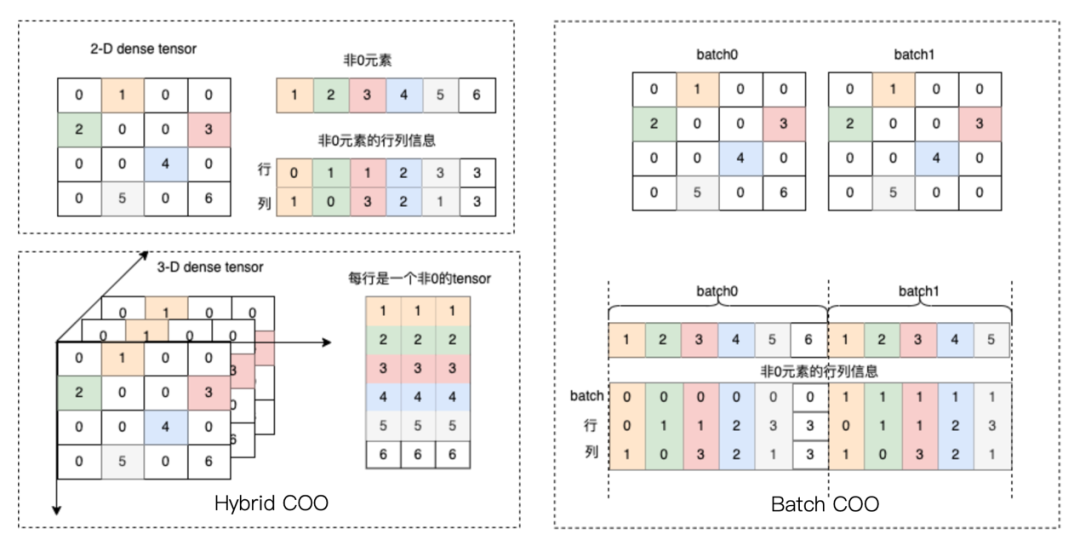``indices = [[0, 1, 1, 2, 3, 3],            [1, 0, 3, 2, 1, 3]]values = [1, 2, 3, 4, 5, 6]dense_shape = [4, 4]coo = paddle.sparse.sparse_coo_tensor(indices, values, dense_shape)print(coo)# Tensor(shape=[4, 4], dtype=paddle.int64, place=Place(gpu:0), stop_gradient=True, #        indices=[[0, 1, 1, 2, 3, 3],#                 [1, 0, 3, 2, 1, 3]], #        values=[1, 2, 3, 4, 5, 6])``

CSR稀疏格式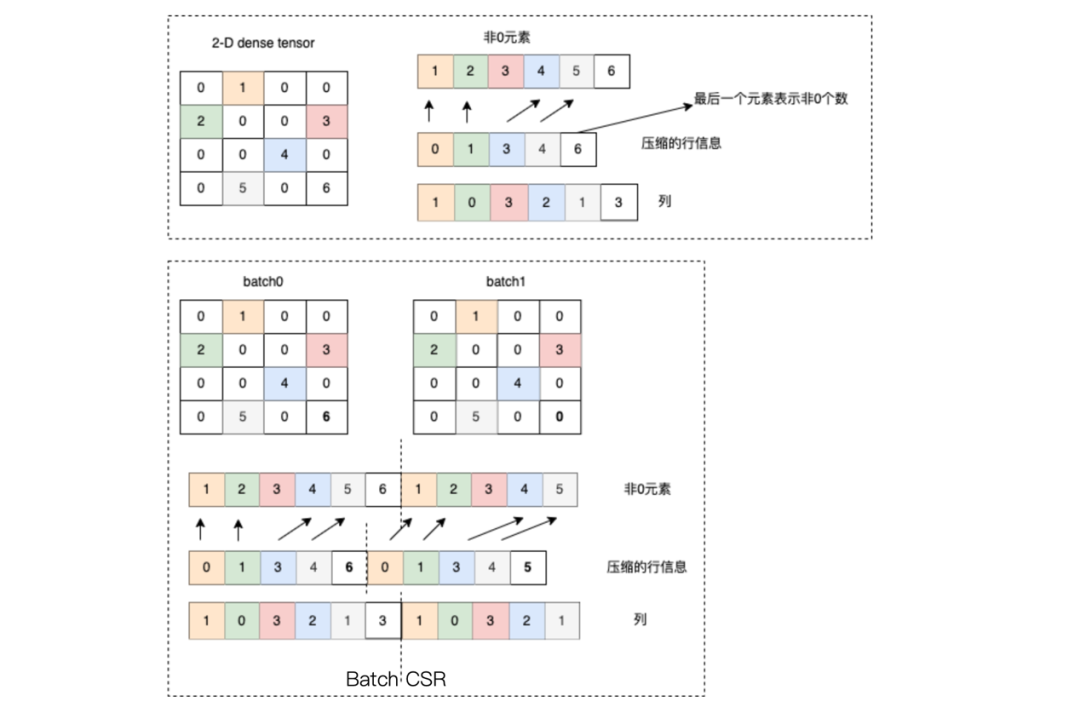``crows = [0, 1, 3, 4, 6]cols = [1, 0, 3, 2, 1, 3]values = [1, 2, 3, 4, 5, 6]dense_shape = [4, 4]csr = paddle.sparse.sparse_csr_tensor(crows, cols, values, dense_shape)print(csr)# Tensor(shape=[4, 4], dtype=paddle.int64, place=Place(gpu:0), stop_gradient=True, #        crows=[0, 1, 3, 4, 6], #        cols=[1, 0, 3, 2, 1, 3], #        values=[1, 2, 3, 4, 5, 6])``

``dense = paddle.to_tensor([[0, 1, 0, 2],                           [0, 0, 3, 4]], dtype='float32')coo = dense.to_sparse_coo(sparse_dim=2)# Tensor(shape=[2, 4], dtype=paddle.float32, place=Place(gpu:0), stop_gradient=True, #       indices=[[0, 0, 1, 1],#                [1, 3, 2, 3]], #       values=[1., 2., 3., 4.])csr = dense.to_sparse_csr()# Tensor(shape=[2, 4], dtype=paddle.float32, place=Place(gpu:0), stop_gradient=True, #       crows=[0, 2, 4], #       cols=[1, 3, 2, 3], #       values=[1., 2., 3., 4.]）``

4

``import paddledef func(x):    return paddle.dot(x, x)x0 = paddle.to_tensor([1.3, 2.7])results = paddle.incubate.optimizer.functional.minimize_bfgs(func, x0)print("is_converge: ", results)print("the minimum of func is: ", results)# is_converge:  is_converge:  Tensor(shape=, dtype=bool, place=Place(gpu:0), stop_gradient=True,#        [True])# the minimum of func is:  Tensor(shape=, dtype=float32, place=Place(gpu:0), stop_gradient=True,#        [0., 0.])``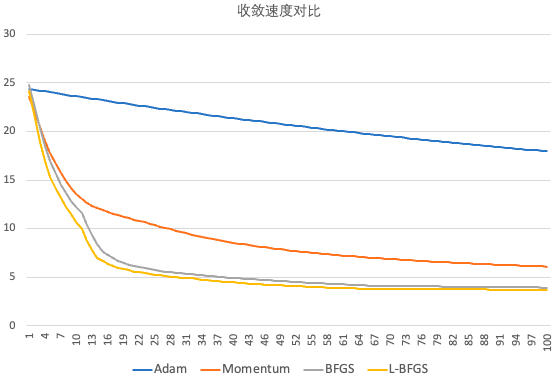5

（1）框架性能分析API

（2）硬件设备管理API

• max_memory_allocated﻿
• max_memory_reserved﻿
• ﻿memory_allocated﻿和﻿memory_reserved﻿

（3）视觉和语音领域高层API

6